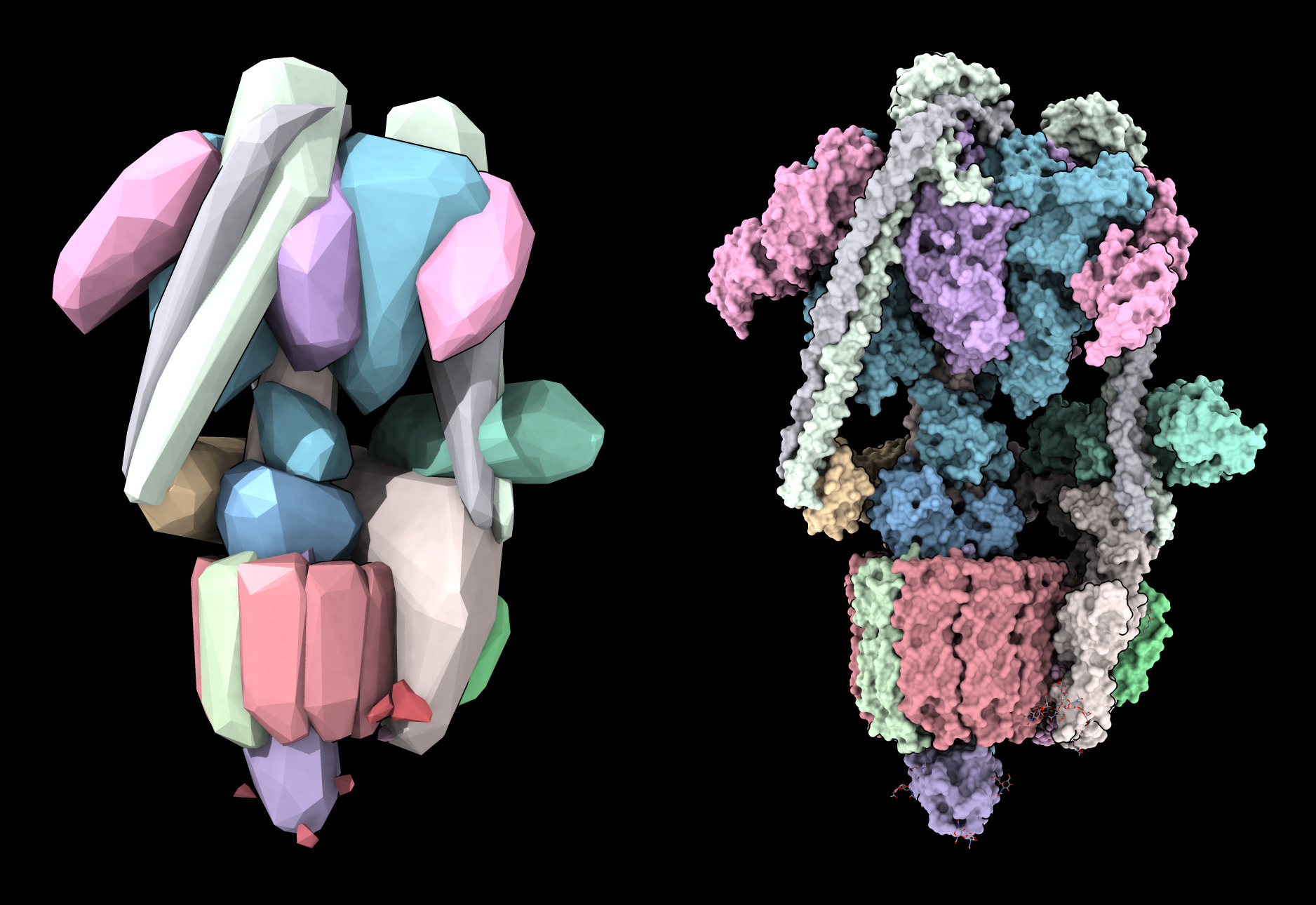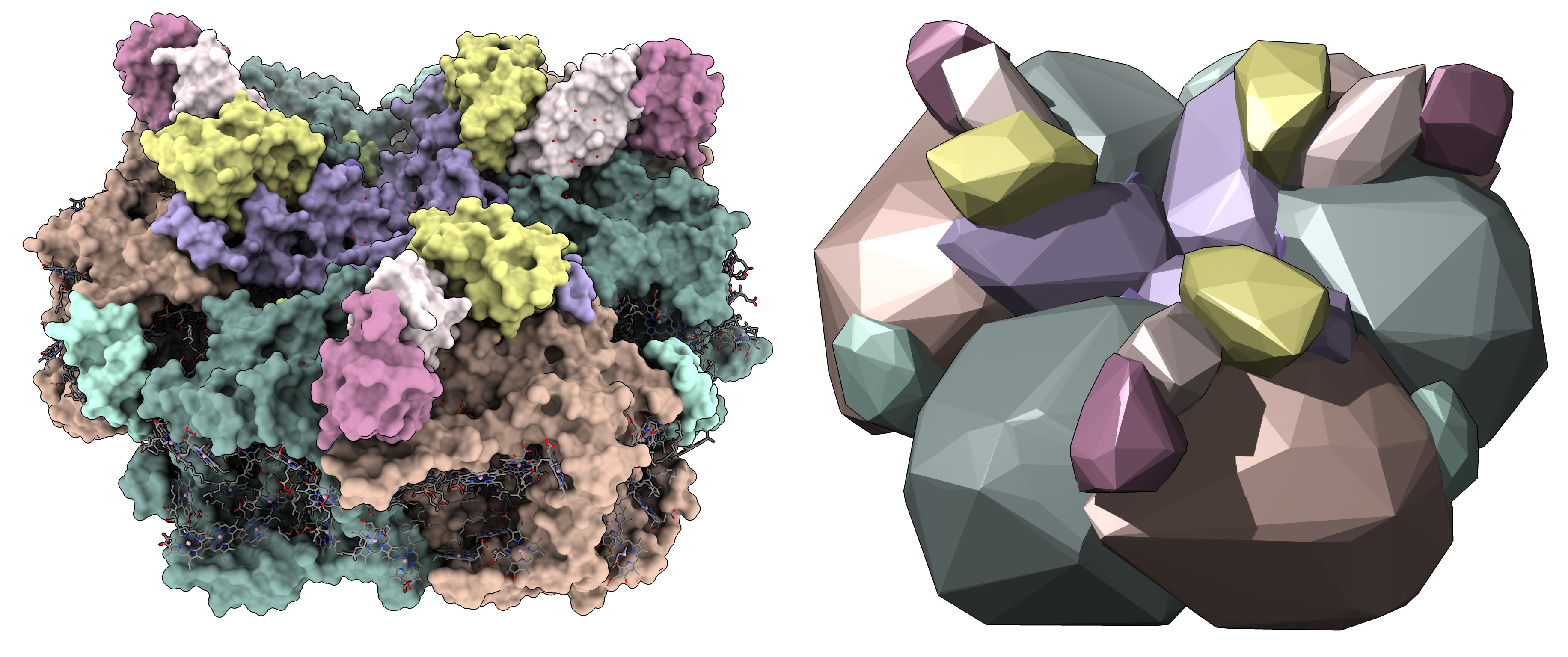# Convex hull of atoms

Here is Python code defining a ChimeraX command to show the convex hull of atoms as a surface.

`````` open convex_hull.py
open 6wm2
convexhull #1 sharp true eachChain true
``````

It gives the human V-ATPase assembly a mineral appearance and uses very few triangles (5000 for the convex hull in this example, versus 9 million for a solvent excluded molecular surface). The option “sharp true” makes the edges between the triangular facets sharp. The “eachChain true” option make a convex hull surface for each chain instead of a single convex hull surface for all the atoms.Another example showing photosystem I, PDB 6kmx.Here is the Python code convex_hull.py

```    #
# Make a convex hull surface for a set of atoms.
#
def convex_hull(session, atoms, sharp = False, mesh = False, color = None,
each_chain = False, name = None, open = True):
'''
Make a surface spanning the convex hull from the atom center positions.
'''
if each_chain:
surfs = []
for struct, chain_id, chain_atoms in atoms.by_chain:
from numpy import uint8
c = chain_atoms.colors.mean(axis=0).astype(uint8) if color is None else color
s = convex_hull(session, chain_atoms, sharp=sharp, mesh=mesh, color=c,
name = chain_id, open=False)
surfs.append(s)
if open:
session.models.add_group(surfs, name = f'Convex hull {len(surfs)} chains')
return surfs

vertices = atoms.scene_coords
from scipy.spatial import ConvexHull
c = ConvexHull(vertices)
triangles = c.simplices
orient_facets(vertices, triangles)

if sharp:
# Duplicate vertices for every triangle so each
# triangle vertex gets its own normal vector.
vertices = vertices[triangles.flat]
n = len(triangles)
from numpy import arange, int32
triangles = arange(3*n, dtype = int32).reshape((n,3))

# Compute normal vectors for lighting.
from chimerax.surface import calculate_vertex_normals
normals = calculate_vertex_normals(vertices, triangles)

if name is None:
name = f'Convex hull of {len(atoms)} atoms'
from chimerax.core.models import Surface
s = Surface(name, session)
s.set_geometry(vertices, normals, triangles)
s.display_style = s.Mesh if mesh else s.Solid
s.clip_cap = True  # Cover holes when clipping
if color is not None:
s.color = color
if open:

return s

def orient_facets(vertices, triangles):
'''
Change the triangles to have consistent vertex order for outward normals.
Unfortunately the vertex order for each triangle is random.
We need them all oriented outward to produce outward normal vectors.
'''
c = vertices.mean(axis = 0)        # Center point
from numpy import cross, dot
for t in triangles:
v0,v1,v2 = vertices[t]
if dot(v0-c, cross(v1-v0, v2-v0)) < 0:
t,t = t,t

def register_command(session):
from chimerax.core.commands import CmdDesc, register, FloatArg, IntArg, BoolArg, Color8Arg, StringArg
from chimerax.atomic import AtomsArg
desc = CmdDesc(required=[('atoms', AtomsArg)],
keyword=[('sharp', BoolArg),
('mesh', BoolArg),
('color', Color8Arg),
('name', StringArg),
('each_chain', BoolArg),
('open', BoolArg)],
synopsis='Show the convex hull of a set of atoms as a surface')
register('convexhull', desc, convex_hull, logger=session.logger)

register_command(session)
```

Tom Goddard, October 10, 2022